# C274 – ISO 5167 Sizing Calculation

## Description

This calculates the flow element size of various differential pressure based flow meters according to the ISO 5167 technical standard.

ISO 5167: Measurement of fluid flows by means of pressure differential devices inserted in circular cross-section conduits running full

ISO TR9464: Guidelines for the use of ISO 5167

BS1042: Part 1. Pressure differential devices – Section 1.2 Specification for square-edged orifice plates and nozzles (with drain holes, in pipes below 50mm diameter, as inlet and outlet devices) and other orifice plates and Borda inlets (1984)

24509-54 – Flow Calculations for the V-Cone and Wafer-Cone Flow meters – McCrometer

24517-16 – V-Cone Flow Meter Technical Brief – McCrometer

Kelton calculation reference C274

Kelton calculation C270 – ISO 5167 Orifice Mass Flow Calculation

Kelton calculation C271 – ISO 5167 Venturi Mass Flow Calculation

Kelton calculation C272 – Cone Mass Flow Calculation

Kelton calculation C276 – ISO 5167-5:2016 Cone Mass Flow Calculation

## Options

### Meter Type

• Orifice
• Venturi
• V-Cone
• Wafer-Cone
• Cone (ISO 5167-5)

This option group allows selection of the meter type that the sizing calculation is to be performed on.

### Standard version

Allows user to select from different revisions of the standard being applied.

### Fluid

• Gas
• Liquid

This option allows selection of whether the fluid in question is a liquid or a gas.

### Expansibility factor

• User entered
• Calculated

This option give the choice of calculating expansibility or entering a known value when the fluid is a gas.

### Tapping Type

• Flange
• D/D2
• Corner

This option group allows the selection of the tapping type used for the pressure measurements on the orifice plate system.

### Drain Hole

• None
• BS 1042

This option allows for selection of a drain hole correction according to BS 1042.

### Discharge coefficient

• User entered
• Variable with Reynold’s number
• Classical venturi with ‘as cast’ convergent section
• Classical venturi with rough-welded convergent section
• Classical venturi with a machined convergent section
• Venturi nozzle
• Uncalibrated Cone

This option allows the user to choose how discharge coefficient will be determined for a venturi tube. If a cone meter is selected only the first two options will be available for selection.

### Exit cone angle

• 15°

This option determines the equation used to calculate the permanent pressure loss associated with a venturi.

## Calculation

### Sizing Calculation

The orifice bore and venturi throat diameter are related to the diameter of the piping in the equation: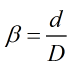For cone meters the relationship is as follows: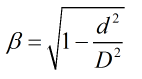Where d = Flow element diameter = Pipe diameter

The following relationships are applied in an iterative loop to solve for the flow element diameter at operating temperature.

For an orifice plate: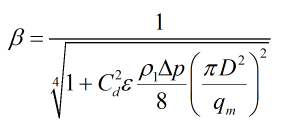For a venturi tube: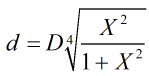For V-cones and Wafer-cones: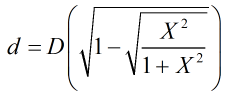In these equations X is calculated by: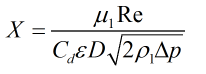Where D = Pipe diameter = Differential pressure = Upstream density = Discharge coefficient = Expansibility factor = Dynamic viscosity = Reynold’s number = Mass flowrate

A temperature correction is then made to calculate the size of the flow element diameter at calibration temperature: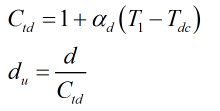Where T1 = Upstream temperature = Calibration temperature of the flow element = Thermal expansion coefficient of flow element

If a drain hole needs to be taken into account, orifice bore is calculated iteratively by employing a root finding algortihm around the equation: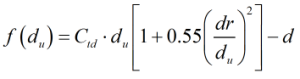Where dr = Drain hole diameter = Orifice bore at calibrated temperature = Orifice bore corrected for operating temperature = Temperature correction

Back to FLOCALC Calculations